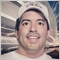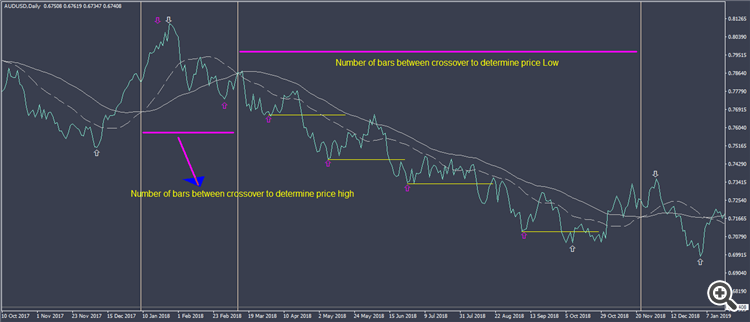# How to get no.of.bars between fast moving average above slow moving average30

I tried get the bars between two ma crossed but it not reset and start from 0 it's looping ever please guide me in right direction167

metapark:
I tried get the bars between two ma crossed but it not reset and start from 0 it's looping ever please guide me in right direction

Put the part/function/piece of the code here so I can help you (but not the full code)30```int j,k;

double ma1 = iMA(NULL, PERIOD_CURRENT, MAFast, 0, MODE_SMA, PRICE_CLOSE, 1);
double ma2 = iMA(NULL, PERIOD_CURRENT, MASlow, 0, MODE_SMA, PRICE_CLOSE, 1);
for(int i = 0; i < Bars -1; i++)
{ if (ma1>ma2)
j=i;
print("up trend bar=",j);
else if (ma1>ma2)
k=i;
print("down trend bar=",k);}
}```
Thanks for your kind quick response167

metapark:
Thanks for your kind quick response

The values of maslow,mafast are not defined.30

rrocchi:

The values of maslow,mafast are not defined.

updated code please check rrocchi thanks167

metapark:

updated code please check rrocchi thanks

well,  the comparison is fine...

But you are comparing the same iMA values every-time. The last parameter "1" is the Shift parameter, it means, shift backward "x" amount of bars

`iMA(NULL, PERIOD_CURRENT, MAFast, 0, MODE_SMA, PRICE_CLOSE, 1);`

Considering you are getting the values outside the loop, the values are the same on every loop interaction.

you can test what I am saying by it modifying the print line to:

```print("up trend bar='", j, "' ma1='", ma1, "' ma2="', ma2, "'");

// also change the other print line to:

print("down trend bar=",k, " ma1=", ma1, " ma2=", ma2);```

Run again and check the print results after modifying the print lines

Also there you need to to determine the scope of the if comparison by using delimiters { } because you have 2 commands inside the if condition:

Code fixed for this situation:

```int j,k;

double ma1 = iMA(NULL, PERIOD_CURRENT, MAFast, 0, MODE_SMA, PRICE_CLOSE, 1);
double ma2 = iMA(NULL, PERIOD_CURRENT, MASlow, 0, MODE_SMA, PRICE_CLOSE, 1);

for(int i = 0; i < Bars -1; i++) {

if (ma1>ma2) {
j=i;
print("up trend bar=",j);
}

else if (ma1>ma2) {
k=i;
print("down trend bar=",k);
}

}

```

But it sill has another problem, because you are comparing the same values (as I mentioned) so you have to change the last parameter of iMA from 1 to i:

```
int j,k;

double ma1 = iMA(NULL, PERIOD_CURRENT, MAFast, 0, MODE_SMA, PRICE_CLOSE, 1);
double ma2 = iMA(NULL, PERIOD_CURRENT, MASlow, 0, MODE_SMA, PRICE_CLOSE, 1);

for(int i = 0; i < Bars -1; i++) {

ma1 = iMA(NULL, PERIOD_CURRENT, MAFast, 0, MODE_SMA, PRICE_CLOSE, i);
ma2 = iMA(NULL, PERIOD_CURRENT, MASlow, 0, MODE_SMA, PRICE_CLOSE, i);

if (ma1>ma2) {
j=i;
print("up trend bar=", j, " ma1=", ma1, " ma2=", ma2);
}

else {
k=i;
print("down trend bar=",k, " ma1=", ma1, " ma2=", ma2);
}

}```

Try it please. (I wrote this here on the forum, have not tested but it should be OK)30

rrocchi:

well,  the comparison is fine...

But you are comparing the same iMA values every-time. The last parameter "1" is the Shift parameter, it means, shift backward "x" amount of bars

Considering you are getting the values outside the loop, the values are the same on every loop interaction.

you can test what I am saying by it modifying the print line to:

Run again and check the print results after modifying the print lines

Also there you need to to determine the scope of the if comparison by using delimiters { } because you have 2 commands inside the if condition:

Code fixed for this situation:

But it sill has another problem, because you are comparing the same values (as I mentioned) so you have to change the last parameter of iMA from 1 to i:

Try it please. (I wrote this here on the forum, have not tested but it should be OK)

thanks for code mate but still issue getting similar loop not breaking once crossed it continue to from up trend bar 72 to downd trend bar 73 instead 1167

metapark:
thanks for code mate but still issue getting similar loop not breaking once crossed it continue to from  up trend bar 72 to downd trend bar 73 instead 1

Can you post the log of the "print" messages, please?30

rrocchi:

Can you post the log of the "print" messages, please?

Here you go mate unzip using 7zip please

Files:
20190829.zip 781 kb